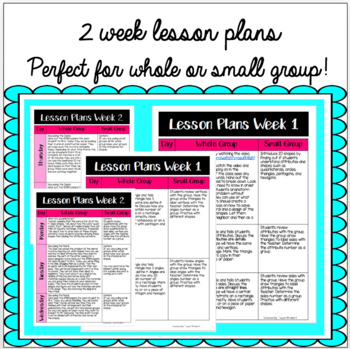# Stuck on STEM Problem-Based Learning Unit on ShapesSubject
Resource Type
File Type
PDF (1 MB|26 pages)
Standards
\$4.50
• Product Description
• Standards
This packet includes:
- Two week lesson plans - day by day of whole group and small group lesson ideas
- Parent letter for STEM materials request - filled in and a blank copy
- 2-part word problem for math and STEM
- Worksheet supports the breakdown of the word problem for math
- Math worksheet for 2D shapes
- Math worksheet for attributes
- Math worksheet for sides
- Math worksheet for angles
- Math worksheet for vertices
- STEM Activity Guidelines
- STEM Process page in color
- STEM Process page in black and white

If you like this, you may be interested in:
Life Cycle Unit BUNDLE
Stuck on STEM Measurement
Stuck on STEM Money

If you like this product, remember to click "follow me" to be one of the first to hear when I post a new product!
Partition shapes into parts with equal areas. Express the area of each part as a unit fraction of the whole. For example, partition a shape into 4 parts with equal area, and describe the area of each part as 1/4 of the area of the shape.
Partition a rectangle into rows and columns of same-size squares and count to find the total number of them.
Partition circles and rectangles into two and four equal shares, describe the shares using the words halves, fourths, and quarters, and use the phrases half of, fourth of, and quarter of. Describe the whole as two of, or four of the shares. Understand for these examples that decomposing into more equal shares creates smaller shares.
Use addition to find the total number of objects arranged in rectangular arrays with up to 5 rows and up to 5 columns; write an equation to express the total as a sum of equal addends.
Apply properties of operations as strategies to add and subtract. If 8 + 3 = 11 is known, then 3 + 8 = 11 is also known. (Commutative property of addition.) To add 2 + 6 + 4, the second two numbers can be added to make a ten, so 2 + 6 + 4 = 2 + 10 = 12. (Associative property of addition.)
Total Pages
26 pages